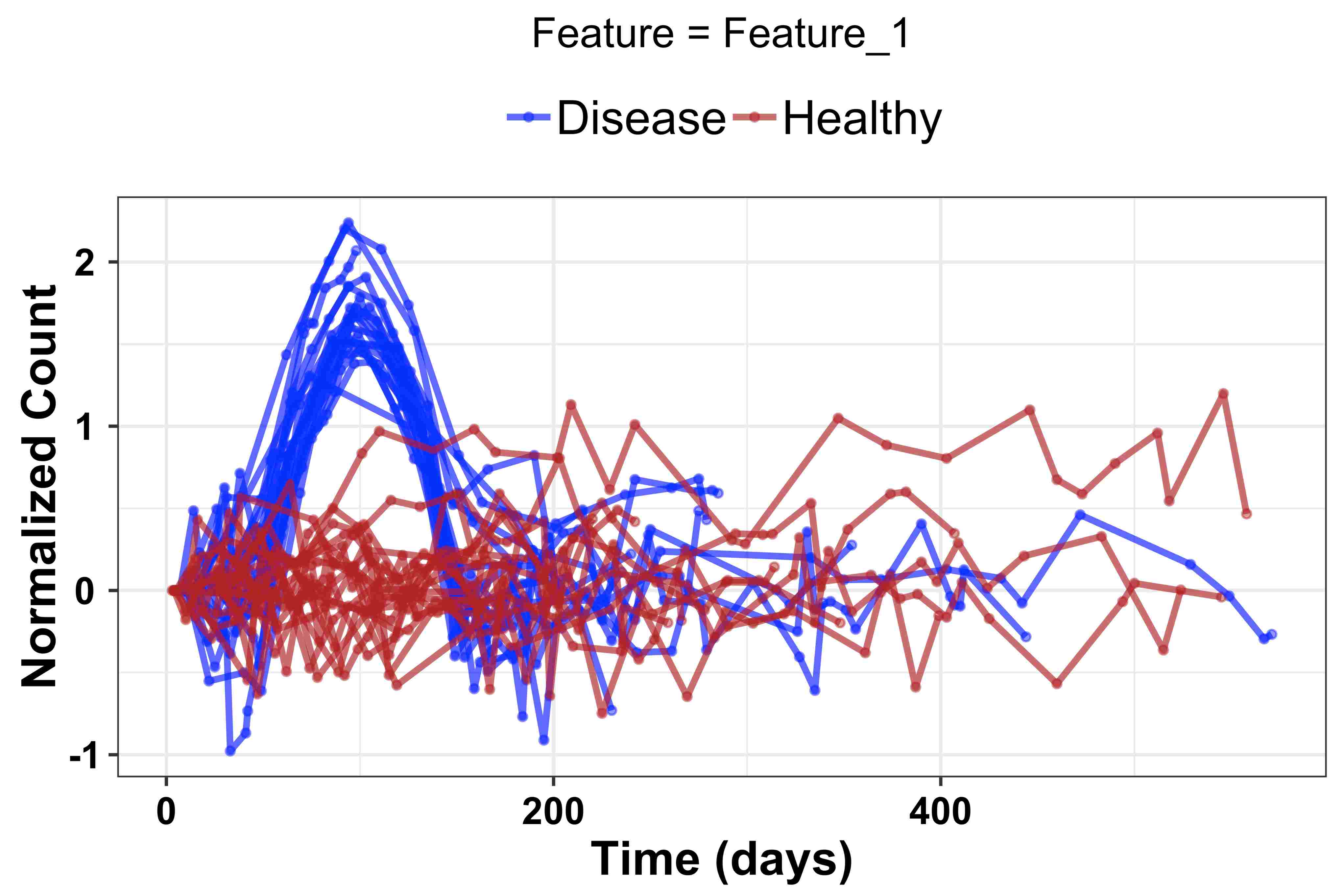# An Introduction to the OmicsLonDA Package

#### 2021-05-19

OmicsLonDA (Omics Longitudinal Differential Analysis) is a statistical framework that provides robust identification of time intervals where omics features are significantly different between groups. OmicsLonDA is based on 5 main steps:

1. Adjust measurements based on each subject’s specific baseline
2. Global testing using linear mixed-effect model to select candidate features and covariates for time intervals analysis
3. Fitting smoothing spline regression model
4. Monte Carlo permutation to generate the empirical distribution of the test statistic
5. Inference of significant time intervals of omics features.

## Getting Started

• R(>= 3.6)

### Installation

``````if (!requireNamespace("BiocManager", quietly = TRUE))
install.packages("BiocManager", repos = "http://cran.us.r-project.org")
BiocManager::install("OmicsLonDA")``````

### Example

``````library(OmicsLonDA)
library(SummarizedExperiment)

data("omicslonda_data_example")``````

The measurment matrix represents count/intensity of features from an omic experiment. Columns represent various samples from different subjects longitudinally. Rows represent various features. Here is an example:

``````omicslonda_data_example\$ome_matrix[1:5, 1:5]
#>           Sample_2  Sample_3  Sample_4  Sample_5 Sample_6
#> Feature_1 13.84393  7.976260  8.392602  7.511995 25.05878
#> Feature_2 17.35090 12.151211 12.758727 11.825749 28.55361
#> Feature_3 17.61570 13.617460 11.505888 13.906188 29.41523
#> Feature_4 17.63444 11.557786 11.081131 12.852518 28.61033
#> Feature_5 18.49954  9.642724 12.765664 11.103249 29.09817``````

The metadata dataframe contains annotations for each sample. Most impotantly it should have at least: (a) “Subject”: which denote from which subject this sample is coming from, (b) “Group”: which represents which group this sample is from (eg., healthy, disease, etc), (c) “Time”: which represents the collection time of the corresponding sample. Here is an example:

``````head(omicslonda_data_example\$metadata)
#>          Subject   Group Time
#> Sample_2    SA_1 Disease   11
#> Sample_3    SA_1 Disease   22
#> Sample_4    SA_1 Disease   40
#> Sample_5    SA_1 Disease   49
#> Sample_6    SA_1 Disease   69
#> Sample_7    SA_1 Disease   75``````

## Create SummarizedExperiment object

``````se_ome_matrix = as.matrix(omicslonda_data_example\$ome_matrix)
omicslonda_se_object = SummarizedExperiment(assays=list(se_ome_matrix),

## Adjust for baseline using CLR

``omicslonda_se_object_adjusted = adjustBaseline(se_object = omicslonda_se_object)``

``````assay(omicslonda_se_object_adjusted)[1:5, 1:5]
#>           Sample_2   Sample_3   Sample_4   Sample_5  Sample_6
#> Feature_1        0 -0.5513775 -0.5004965 -0.6113461 0.5933772
#> Feature_2        0 -0.3562154 -0.3074287 -0.3833650 0.4981392
#> Feature_3        0 -0.2574379 -0.4259317 -0.2364567 0.5127220
#> Feature_4        0 -0.4224943 -0.4646099 -0.3163138 0.4839143
#> Feature_5        0 -0.6515421 -0.3709867 -0.5105080 0.4529296``````

## Visualize first feature

``````omicslonda_test_object = omicslonda_se_object_adjusted[1,]
visualizeFeature(se_object = omicslonda_test_object, text = "Feature_1",
unit = "days", ylabel = "Normalized Count",
col = c("blue", "firebrick"), prefix = tempfile())``````Visualize first feature

## Specify interval bounds

``points = seq(1, 500, length.out = 500)``

## Run OmicsLonDA on the first feature

``````res = omicslonda(se_object = omicslonda_test_object, n.perm = 10,
fit.method = "ssgaussian", points = points, text = "Feature_1",
parall = FALSE, pvalue.threshold = 0.05,
adjust.method = "BH", time.unit = "days",
ylabel = "Normalized Count",
col = c("blue", "firebrick"), prefix = tempfile())``````

## Visualize fitted spline of the first feature

``````visualizeFeatureSpline(se_object = omicslonda_test_object, omicslonda_object = res, fit.method = "ssgaussian",
text = "Feature_1", unit = "days",
ylabel = "Normalized Count",
col = c("blue", "firebrick"),
prefix = "OmicsLonDA_example")``````